# Euclid : Book 1 : Definition 9

The Elements of Euclid - Part 1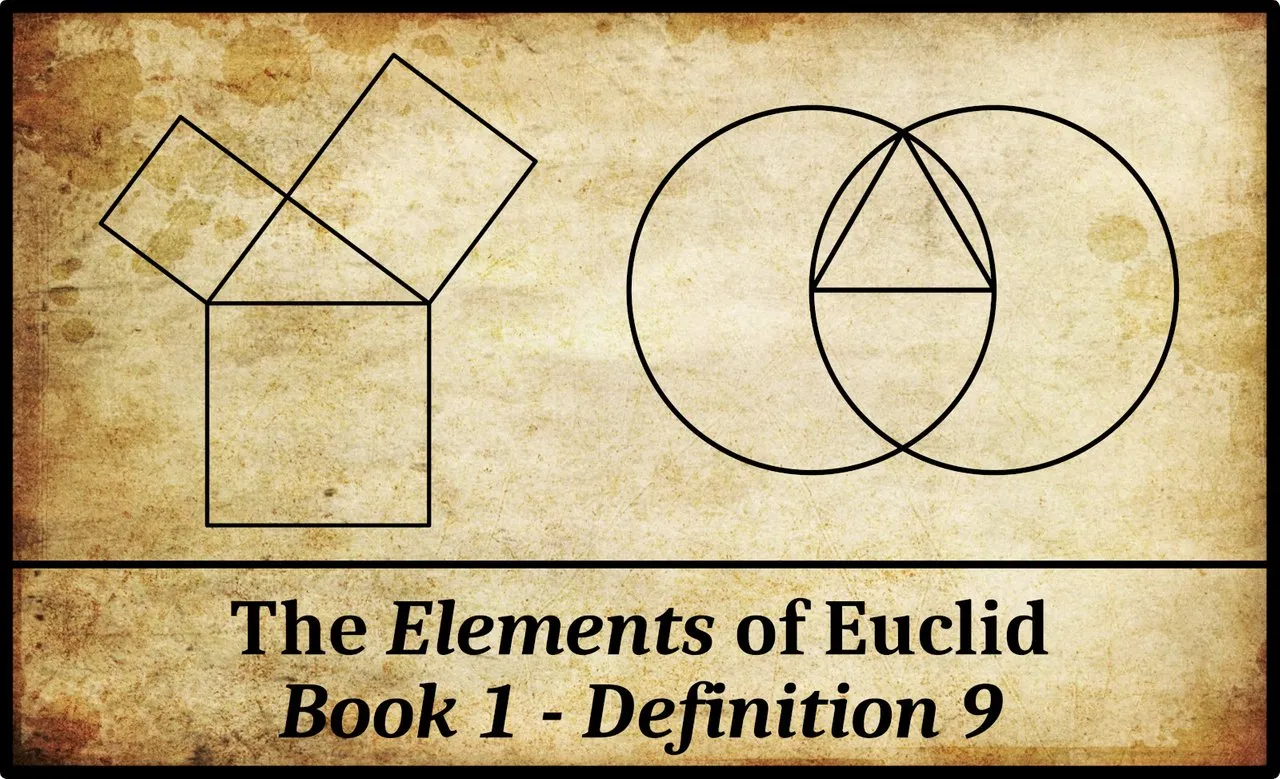In Book 1 of Euclid’s Elements, Definition 9 reads (Fitzpatrick 6):

GreekEnglish
θʹ. Ὄταν δὲ αἱ περιέχουσαι τὴν γωνίαν γραμμαὶ εὐθεῖαι ὦσιν, εὐθύγραμμος καλεῖται ἡ γωνία.9. And when the lines containing the angle are straight then the angle is called rectilinear.

Some editions of Euclid include the word εἰρημένην [eirēmenēn] between τὴν and γωνίαν, so that the passage reads:

And when the lines containing the said angle ...

François Peyrard’s includes this in his French edition of 1814, which is based on the 10th-century Codex Vat.gr.190.pt.1 in the Vatican Library (Peyrard 2, 453). In fact, εἰρημένην has been circled in pencil in this manuscript—by Peyrard? (It is also misspelt εἰρημέμην [eirēmemēn].) Codex 190 is, however, the only early manuscript that includes this extra word (Heiberg 3):Vaticanus Graecus 190, Part 1, Folio 14r (Definition 9)

Definition 8 has just defined a plane angle in terms of the inclination of two lines that lie in the plane and meet one another. But, as we have seen, for Euclid the term line includes what we would call a curve. Thus Definition 8 covers not only angles as we think of them today but also the so-called mixed and curvilinear angles. These include the horn angle between a circle and one of its tangents, which is the subject of Proposition 3:16.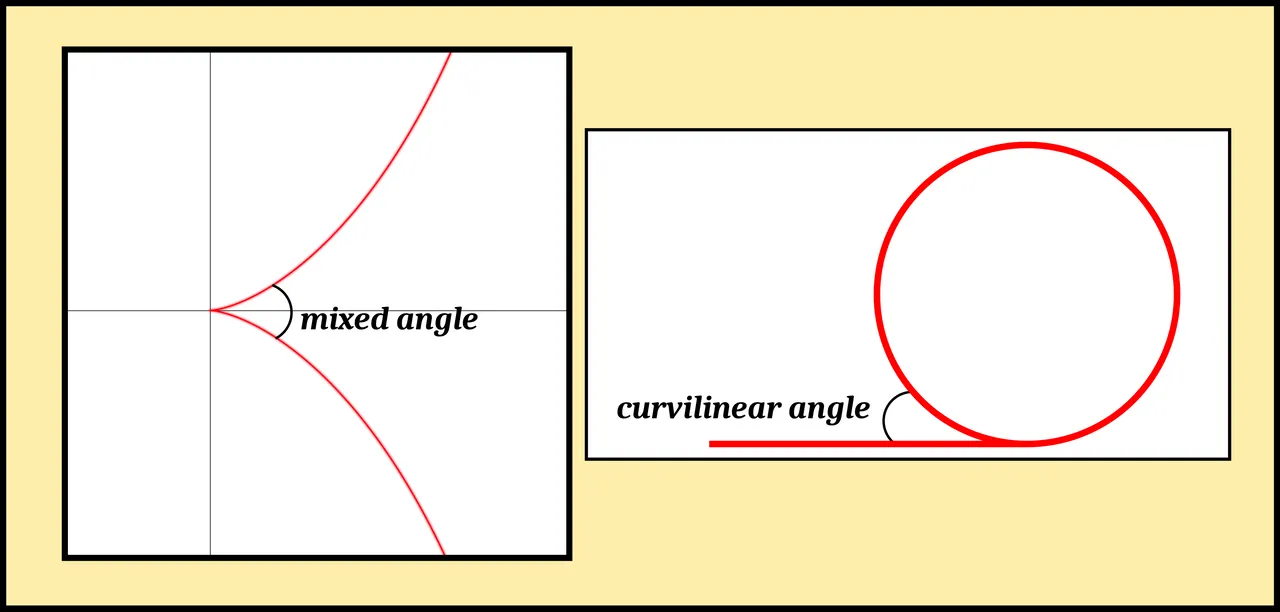A Mixed Angle and a Curvilinear Angle

Definition 9 restricts the term rectilinear angle to those angles which are created by two straight lines—in other words, angles as we usually think of them today.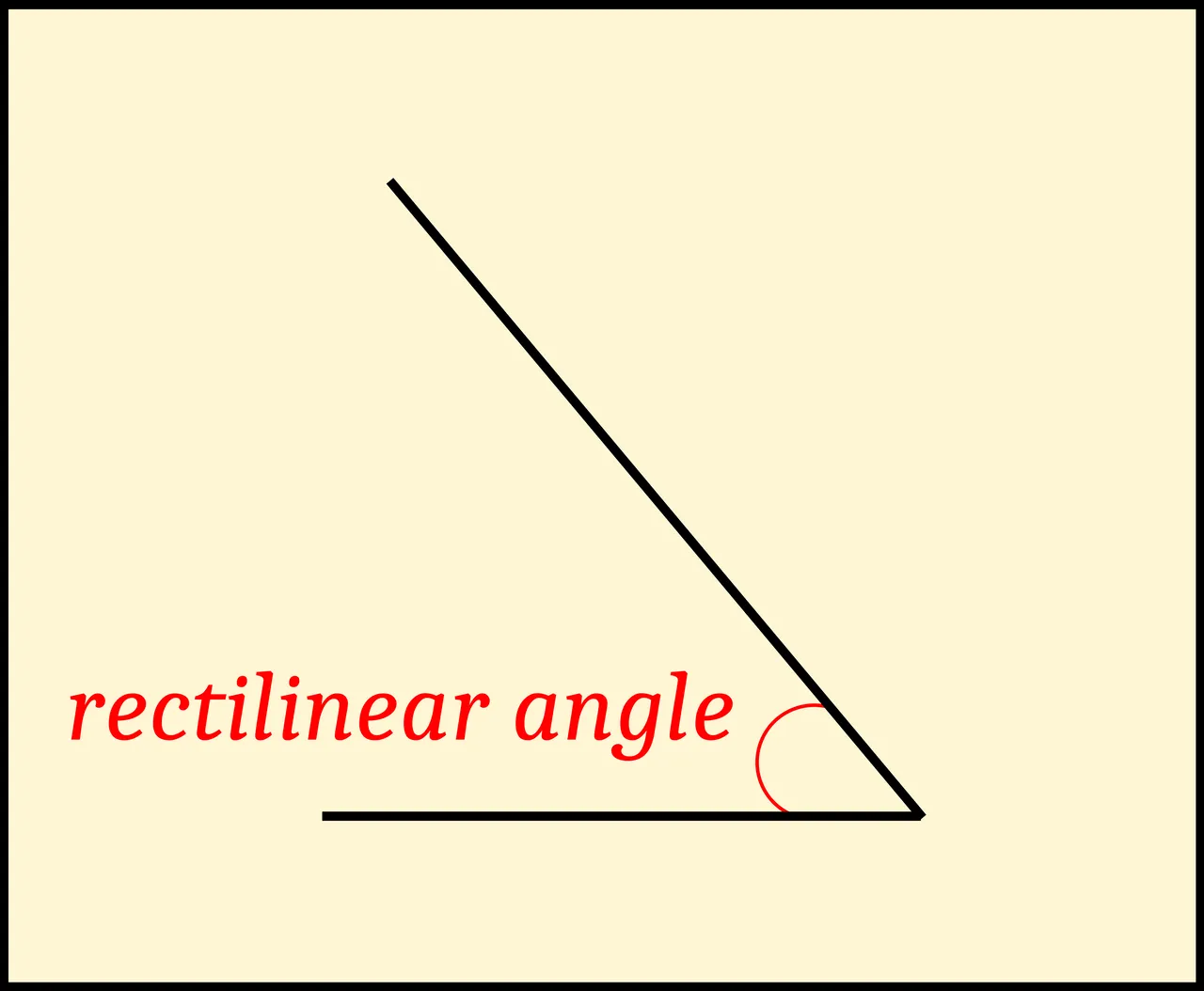A Rectilinear Angle

Thomas Heath translates Euclid’s εὐθύγραμμος [euthugrammos] as rectilineal (Heath 176). The ancient Greek word which Euclid uses for this technical term is also the modern Greek for rectilinear. In Liddell & Scott’s Greek-English Lexicon, the term is hyphenated:εὐθύ-γραμμος (Scott & Liddell 601)

# Magnitudes and Measurements

In Euclid’s Elements, an important distinction is made between the magnitude of a rectilinear angle and its measurement. The latter is characterized by a number, but the magnitude of an angle is, as it were, a pre-numerate concept. In terms of magnitudes, one angle may be greater than, less than, or equal to another angle. In other words, angular magnitudes can be compared to one another, but without the metrical concept of numerical measurement we cannot be more specific than this. We cannot say, for example, that one angle is twice as big as another angle, or half as big.

In Euclid, the magnitudes of rectilinear angles can even be added together, though this addition is very different from arithmetic addition. For example, in Proposition 1:32, Euclid proves a familiar theorem: In any triangle, if one of the sides is produced then the external angle is equal to the sum of the two internal and opposite angles. He does not say anything about the measurements of these angles, simply that the magnitude of the external angle is equal to the magnitude one would get if one were to combine the two internal opposite angles into a single continuous angle.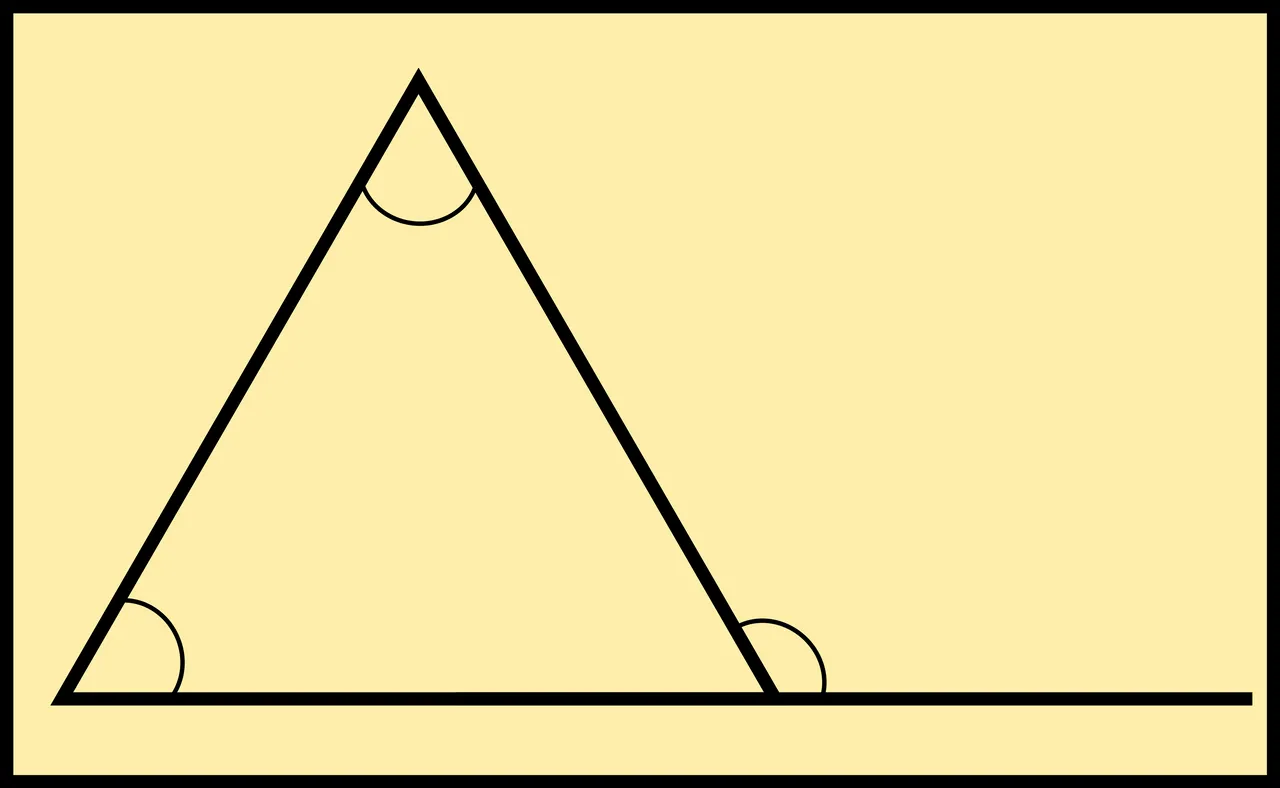The Elements: Proposition 1:32

The arithmetic of angular magnitudes is introduced by Euclid in Proposition 1:13. We will revisit this concept when we come to discuss that proposition.

In the Elements, the only rectilinear angles whose numerical measurements are considered are the right-angle and the straight angle. In Euclid’s day, metrical units for measuring angles—such as degrees and radians—had not yet been introduced. Instead, Euclid defines right-angle as the metrical unit for measuring the rectilinear angle between two lines that are perpendicular to each other. This is, in fact, the purpose of Definition 10. He later uses the metrical unit two right-angles to measure straight angles (eg Propositions 1:13-15).

Thus, in Proposition 1:32, where Euclid also demonstrates that the sum of the three internal angles of a triangle is 180°, he expresses this by saying: the three internal angles of the triangle are equal to two right-angles. This is a numerical equation: the sum of three numbers equals another number. But it also implies that the “sum” of the magnitudes of the three internal angles is equal to the magnitude of a straight angle.

# Proclus

In his Commentary on the First Book of Euclid’s Elements, the 5th-century scholar Proclus has a curiously cryptic comment on this definition. Far from discussing the mathermatical or geometrical implications of Euclid’s definition of a rectilineal angle, he embarks on a short but obscure discussion of Pythagorean symbolism and theology: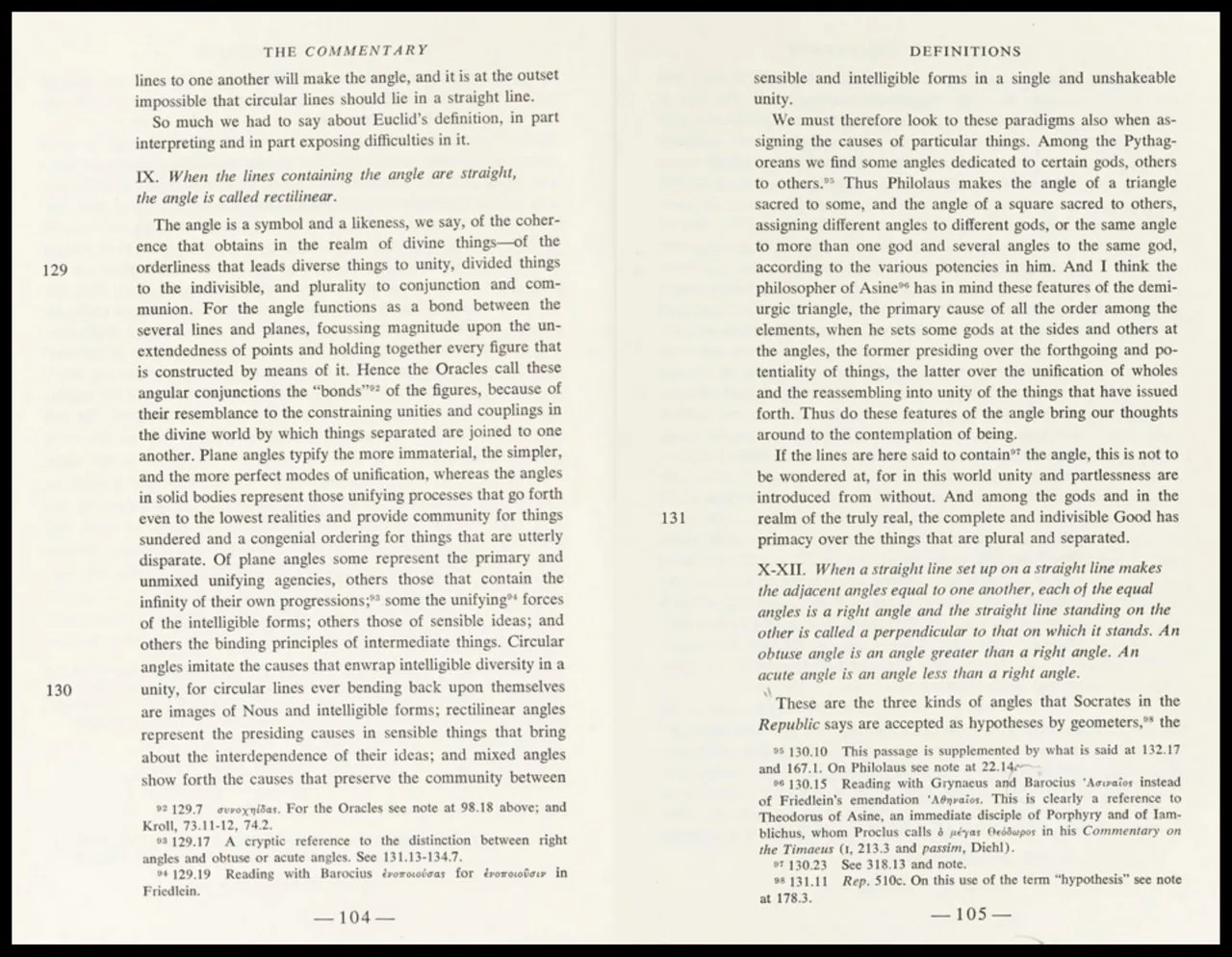Proclus on Euclid, Book 1, Definition 9 (Morrow 104-105)

I leave the reader to make of this what he or she will.

And that’s a good place to stop.

## Online Resources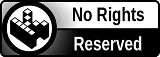H2
H3
H4
3 columns
2 columns
1 column
Join the conversion now